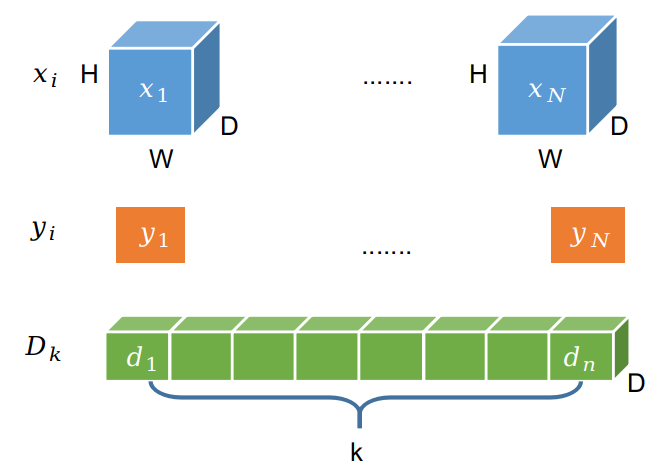# 论文阅读之Interpretable and Accurate Fine-Grained Recognition via Region Grouping

## 实施方法

• Beta 分布：可以理解为概率的概率分布，比如说，知晓了一个事情发生的大致概率，对于接下来发生的事件进行概率预测时，就可以基于这个我们已经知道的大概概率进行预估，也就是这篇文章所提出的先验信息。这个方法可以解决因为观测次数不够而导致的可能的预测偏差，也可以随着训练过程提高对参数估计的精度。
• Wasserstein Distance：可以理解为相同向量空间中，两个不同的分布之间进行转换时需要的“代价”，文中提出的是最小化“部分”寻找到的先验和经验分布的转换代价，文中则是需要估计的经验分布与先验分布的差异。
• U 形分布：直观上看就是两端多中间少，文中认为图像中的特征分布也会类似的情况，即对于特征来说，会呈现概率上的有和无的状态。文章认为图像中的特征的分布是符合 U 形分布的 Beta 分布的，因此设计了正则化方法将特征字典扔到 Wasserstein Distance 中进行度量，这样即可以随着训练的迭代来不断调整特征对应的概率，从而给出在先验“部分”特征出现概率时进一步预测“部分”的重要性。

• “部分”分割：自特征图中将图像分为多个子“部分”区域（以下简称区域）组，记作$$g(\cdot)$$
• 区域特征提取和特征归因发现：使用池化方法提取区域特征并通过给每个特征区域添加注意力向量，记作$$f(\cdot)$$
• 基于注意力的区域分类：对每个向量通过注意力机制计算注意力向量的值，记作$$h(\cdot)$$

• $$X_{1:N}=\{X_n\}$$：表示有 N 个 2D 图片的特征图集合，其中 $$X_{n}\in R^{D\times H\times W}$$ ，表示卷积网络从 $$H\times W$$ 的二维图像中获得的 D 维的特征，是一个维度为 $$D\times H\times W$$ 的矩阵。
• $$y_{1:n}=\{y_n\}$$：表示 N 个图片对应的分类，其中 $$y_n \in [1,...,c]$$ ，需要注意的是这部分的标签只是大类别的标签，并没有细的类别。
• $$D \in R^{D\times K}$$：区域组成“部分”的字典，即有 $$K$$ 种区域，其中 $$D=[d_1,d_2,...,d_K]$$ ，而 $$d_k$$ 则是代表了一个区域类别。
• $$\hat{y}=\phi(X_i,D;\theta)$$：用来分类的决策函数，这个函数在分类时，将特征图 $$X_i$$ 和全局的字典 $$D$$ 都考虑在内， $$\theta$$ 代表优化时的参数，从后续文字可以得知，此部分的优化实际上包括部分区域分割 $$Q$$ 、区域特征提取和归因 $$[Z,a]$$ 以及基于注意力的分类 $$\hat{y}$$ 的函数及对应参数$$\theta_g,\theta_f,\theta_c$$ 。（注：后续对下标$$n$$可能不做具体标注）
• $$Q=g(X,D,;\theta_g)$$: 表示的是区域分配的优化目标，目的是为了通过比对 2D 的特征图 $$X$$ 和区域字典 $$D$$ 生成一个区域分配图 $$Q \in R^{K\times H\times W}$$, 记作 $$g(\cdot)$$
• $$[Z,a]=f(X,Q,D;{\theta}_{f})$$：主要负责了区域特征提取和归因分析，在前一步的区域选择中已经通过区域字典 $$D$$ 搞定了区域分配图 $$Q$$ 之后，这块主要是来得到区域内的特征 $$Z\in R^{D\times K}$$ 并设定一个注意力参数 $$a\in R^K$$来控制所对应区域在最终分类中的重要性，以供后续继续优化，记作 $$f(\cdot)$$
• $$\hat{y}=h(Z,a;\theta_c)$$：表示的是基于注意力的分类，通过将注意力参数 $$a$$ 和区域特征 $$Z$$ 放到线性分类器中，即可通过训练以适应数据，记作 $$h(\cdot)$$但是有个很严肃的问题是，文章认为它需要从弱标签里来学习到对应区域，为了确保“部分”目标的字典可以学习得到，作者假设图片上的特征是从属于一个图片特征集合的，而这个集合即服从 U 形分布，那么这一组特征在图片上可能的概率便可以使用 U 形分布，正则化工作也就是在这部分进行。具体而言，对于给定的一个图片特征集合 $$X_{1:N}$$ ，假设 $$p(d_k|X_{1:N})$$ 是区域 $$d_k$$ 出现在这个集合中的条件概率，并假定 $$p(d_k|X_{1:N})$$ 概率服从 U 形分布，从而可以控制特征是否存在的概率。1。作者在文中并没有做进一步的解释，而是认为对于部分简单的同类图像会包含很多相同的特征，如在小规模的数据集 Cub-200 birds 中，鸟的各个部分特征都是存在的，因此会将这个“特征是否存在”这个事件置为发生，否则对于复杂的数据集，如 ImageNet 中，则置为未发生。

### 部分分割与正则化

#### 区域分配

$q_{ij}^k=\frac{\exp(-\Vert(x_{ij}-d_k)/\sigma_k\Vert_2^2/2)}{\sum_k \exp(-\Vert(x_{ij}-d_k)/\sigma_k\Vert_2^2/2)}$

#### 区域发现的正则化

$W(p(d_k|X_{1:N}),p(d_k|X_{1:N}))=\int_0^1|F^{-1}(z)-\hat{F}^{-1}(z)|dz$

$W(p(d_k|X_{1:N}),p(d_k|X_{1:N}))=\frac{1}{N}\sum_{n=1}^N|\log([\tau_k^*]_i+\epsilon)-\log(\hat{F}^-1(\frac{2n-1}{2N})+\epsilon)|$

### 区域特征提取及归因分析

$z_k^{'}=\frac{1}{\sum_{ij} q_{ij}^k}\sum_{ij}q_{ij}^k(x_{ij}-d_k)/\sigma_k, z_k=\frac{z_k^{'}}{\Vert z_k^{'}\Vert_2}$

### 基于注意力的分类

$\hat{y}=softmax(W f_z(Z)a)$

## 实验效果

1. 这个部分还有一个疑惑就是这个概率分布的事件是什么，即事件的直方图是什么↩︎

2. 目前我还比较疑惑，同时在 CVPR 上公开的版本中，下面所述公式有误，此处已经修正。↩︎

3. 这部分暂时不理解， 但是 $$\epsilon$$ 确实可以避免该值为零↩︎

https://blog.waynehfut.com/2020/10/15/interpretable_recognition_with_group/

Hao Wang

2020年10月15日

2022年7月28日